# Solved Question Paper Chemistry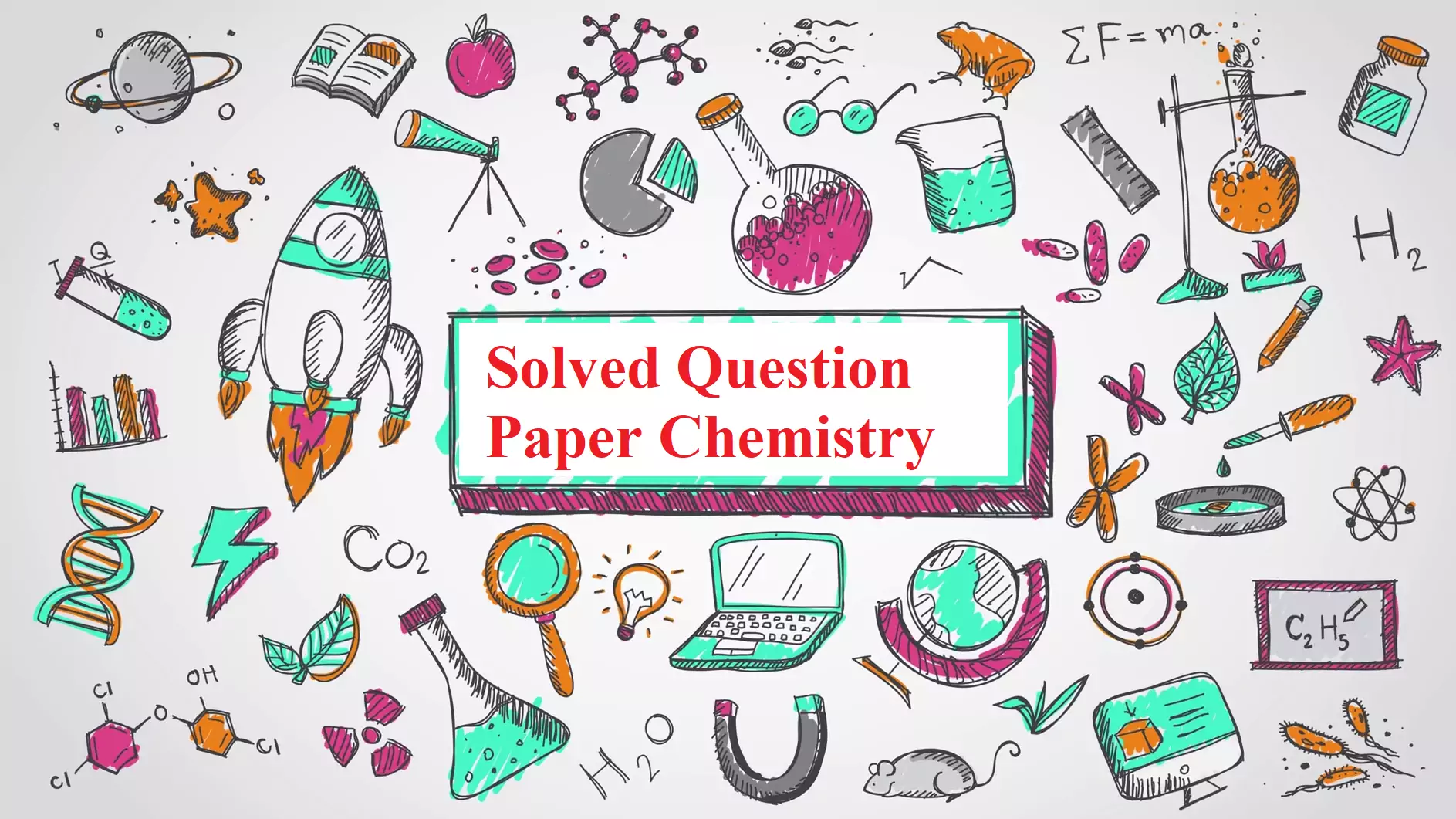Solved Question Paper Chemistry

Class – XII

CHEMISTRY THEORY (043)

Time: 3 Hours                                                                                  M. M.: 70
General Instructions:

1. There are 33 questions in this question paper. All questions are
2. Section A: No. 1 to 16 are objective type questions. Q. No. 1 and 2 are passage-based questions carrying 4 marks each while Q. No. 3 to 16 carry 1 mark each.
3. Section B: Q. No. 17 to 25 are short answer questions and carry 2 marks
4. Section C: Q. No. 26 to 30 are short answer questions and carry 3 marks
5. Section D: No. 31 to 33 are long answer questions carrying 5 marks each.
6. There is no overall choice. However, internal choices have been
7. Use of calculators and log tables is not

SECTION A – (OBJECTIVE TYPE)

The dehydration of phenols and alkylation of phenols by alcohols over thorium were studied at 400–500 °C and atmospheric pressure. Phenol and cresols, when dehydrated gave diaryl ethers as main products. With para-substituted phenols such as p-methoxy,  p-t-butyl,  p-chloro, and p-nitrophenol no ether formation was noticed. All the reactions were accompanied by a considerable amount of coke formation. Alkylation of phenols by alcohols gave a mixture of O- and C-alkylated products under the same reaction conditions. O-alkylation and C-alkylation are parallel reactions.
In addition to acting as an antiseptic, phenol is also a useful precursor in many chemical syntheses to produce pharmaceuticals, food preservatives, polymers, resins and adhesives. Phenolics are also present in a number of biological systems and natural products such as neurotransmitters, flavouring agents, and vitamins to name a few.
The following questions are multiple-choice questions. Choose the most appropriate answer:
(i) What is the IUPAC name of Cresol?
(a) 2-Methylphenol    (b) 3,4 – Dimethylphenol        (c) 2,4 – Dimethylphenol        (d) None of them
Ans i. (a) 2-Methylphenol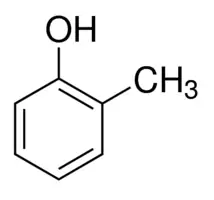(ii) p-Nitrophenol is more acidic due to:-
(a) +R & +I effect                   (b) –R & +I effect
(c) –I & +R effect                   (d) -I & -R effect
Ans ii.  (c) –I & +R effect
Reason: The p- nitrophenol is more acidic than phenol because of the deactivating nature of the nitro group and after losing the protons it makes more resonance stabilization as the nitro group is having strong deactivating nature so it attracts electrons towards it

(iii) When Phenol is reacted with CHCl3 and Aq. KOH it gives:-
(a) Salicylaldehyde                (b)  Crotonaldehyde   (c) Salicyclic acid      (d) Cinnamic acid
Ans iii. (a) Salicylaldehyde(iv) Reaction used to prepare Salicyclic acid from Phenol and CO2 at 5-& atm. is –
(a) Willamson’s reaction                               (b) Kolbe’s reaction
(c) Reimer Tieman reaction                          (d) Friedel – Craft reaction
Ans iv. (b) Kolbe’s reaction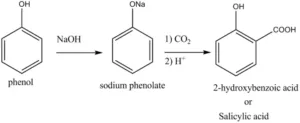Que 2. Read the passage given below and answer the following questions:
A catalyst is an entity that accelerates a chemical reaction without being consumed in the process. This ability is usually referred to as the activity of a catalyst. For a chemical reaction with multiple possible products, a catalyst may promote the production of a specific product, which is referred to as the selectivity of a catalyst. Heterogeneous catalytic reactions occur on the surface of solid catalysts and involve elementary surface chemical processes such as adsorption of reactants from a reaction mixture, surface diffusion and reaction of adsorbed species, and desorption of reaction products.
Chemisorption bonding trends across the periodic table were recognized by using the accumulation of data from adsorption energy measurements. Detailed electronic structures of surface chemical bonds were obtained by spectroscopic techniques.
In these questions (Q. No 5-8), a statement of assertion followed by a statement of reason is given.
Choose the correct answer out of the following choices.
(a) Assertion and reason both are correct statements and reason is the correct explanation for the assertion.
(b) Assertion and reason both are correct statements but the reason is not the correct explanation for the assertion.
(c) Assertion is a correct statement but the reason is the wrong statement.
(d) Assertion is a wrong statement, but the reason is a correct statement.
(i) Assertion – Conversion of SO2 to SO3 is catalysed by V2O5.
Reason – A catalyst accelerates a chemical reaction without being consumed in the process.
Ans (i). b

(ii) Assertion – Heterogeneous catalysis is also known as solid catalysis.
Reason – Most of the reactants adsorb on the surface of catalyst for the reaction.
Ans (ii). a

(iii) Assertion – Selectivity of a catalyst means to promote the production of a specific product out of a number of possible products.
Reason:- The catalyst increases the rate of the reaction without itself undergoing any change.
Ans (iii). b

(iv) Assertion – Heat of adsorption in Chemisorption is higher than physisorption.
Ans (iv). d

OR

Assertion – Adsorption is a surface phenomenon and Absorption is a bulk phenomenon.
Reason –  In Adsorption particles accumulate on the surface and go to the bulk in absorption.
Ans. a

Solved Question Paper Chemistry

Following questions (No. 3 -11) are multiple-choice questions carrying 1 mark each
Que 3. Which of the following option will be the limiting molar conductivity of CH3COOH if the limiting molar conductivity of CH3COONa is 91 Scm2mol-1? Limiting molar conductivity for individual ions are given in the following table.

 S.No Ions limiting molar conductivity / Scm2mol-1 1 H+ 349.6 2 Na+ 50.1 3 K+ 73.5 4 OH- 199.1

a) 350 Scm2mol-1 (b) 375.3 Scm2mol-1 (c) 390.5 Scm2mol-1 (d) 340.4 Scm2mol-1
Ans 3. Limiting molar conductivity
λ0mCH3COOH = λ0mCH3COO + λ0mH+
= λ0mCH3COONa – λ0mNa+ + λ0mH+
= 91 – 50.1 + 349.6 = 390.5 Scm2mol-1

Que 4. The pyrimidine bases present in DNA are
(b) Cytosine and Guanine
(c) Cytosine and Thymine
(d) Cytosine and Uracil
Ans 4. (c) Cytosine and Thymine

OR

The term  anomers of glucose refer to
(a) Isomers of glucose that differ in configurations at carbons one and four (C-1 and C-4)
(b) A mixture of (D)-glucose and (L)-glucose
(c) Enantiomers of glucose
(d) Isomers of glucose that differ in configuration at carbon one (C-1)
Ans 4. (d) Isomers of glucose that differ in configuration at carbon one (C-1)

Que 5. The density of a 2.05 M solution of acetic acid in water is 1.02 g/mL. The molality of the solution
(a) 1.14 mol kg–1                                (b) 3.28 mol kg–1
(c) 0.44 mol kg–1                                (d) 2.28 mol kg–1

Ans 5. Suppose the volume of solution is 1000 ml.
1.02 gm of acetic acid in 1 ml of solution.
1020 gm in 1000 ml of soln.
Number of moles = 2⋅05 M × 1 lit = 2.05 mol
Mass of solute = n × M⋅wt = 2.05 × 60 =123 gm
Mass of solvent =1020 − 123 = 897 gm
Molality =123​ × 1000​ / 60 × 897 =2.28 mol kg−1

Que 6. Which metal in the first series of transition metals exhibits +1 oxidation state most frequently?
(a) Cu                          (b) Sc                          (c) V                                       (d) Ti
Ans 6.  (a) Cu
Reason: Cu metal in the first transition series (3d series) shows +1 oxidation state most frequently. This is because the electronic configuration of Cu is 3d10 4s1 and after losing one electron, it acquires the stable 3d10 fully filled electronic configurations.

OR

Hg is not considered a transition metal due to
(a) Inert pair effect                                         (b) Completely filled 3d subshell
(c) Completely filled 4s subshell                       (d) Common ion effect
Ans. (b) Completely filled 3d subshell.

Que 7. The IUPAC name for the complex [Co(NO2)(NH3)5]Cl2 is
(a) nitrito-N-pentaamminecobalt (III) chloride
(b) nitrito-N-pentaamminecobalt (II) chloride
(c) pentaammine nitrito-N-cobalt (II) chloride
(d) pentammine nitrito-N-cobalt (III) chloride
Ans 7.  (d) pentammine nitrito-N-cobalt (III) chloride

OR

Ambidentate ligands like NO2and SCN are:
(a) Unidentate           (b) Didentate         (c) Polydentate            (d) Has variable denticity
Ans. (a) Unidentate
Reason: Ambidentate ligands are ligands which can bind to the central atom in two places. This is because they have more than one donor atom which can coordinate. It is important to note that these ligands are capable of bonding to a central atom through two different atoms, but only bonds with one of them at a time.

Que 8. Propanamide on reaction with bromine in aqueous NaOH gives
(a) Ethanamine                                                           (b) Propanamine
(c) N-Methyl ethanamine                                             (d) Propanenitrile
Ans 8. (a) Ethanamine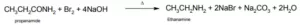Que 9. The coordination number and the oxidation state of the element E in the complex [E(en)2(C2O4)]NO2 are
(a)  6 & 3                             (b) 6 & 2                 (c) 4 & 2                      (d)  4 & 3
Ans 9. (a)  6 & 3

Que 10. Identify B

CH3CH2Br   A   B      “B” is _____.
a) CH3CONH2           (b) CH3Br              (c) CH3COOH              (d) CH3NH2
Ans 10. (c) CH3COOH

Que 11. The fraction of the total volume occupied by the atoms present in a simple cube is:
(a) π / 4                    (b) π / 6                   (c) π3√2                           (d) π4√2
Ans 11. (b) π / 6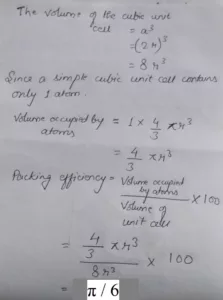Solved Question Paper Chemistry

In the following questions (Q. No. 12 – 16) a statement of assertion followed by a statement of reason is given. Choose the correct answer out of the following choices.

Que 12. Assertion:- Ozone is a powerful oxidizing agent in comparison to oxygen
Reason:- Ozone is diamagnetic but oxygen is paramagnetic
Ans 12. (b)

OR

Assertion:- At room temperature oxygen exist as a diatomic gas whereas sulphur exists as a solid.
Reason:- pπ – pπ multiple Bond in oxygen is more stable
Ans. (a)

Que 13. Assertion: The two strands of DNA are complementary to each other
Reason:-  The hydrogen bonds are formed between specific pairs of bases.
Ans 13. (b)

Que 14. Assertion:- Bond angle in ethers is slightly less than the tetrahedral angle.
Reason:-  There is repulsion between two bulky (-R) groups.
Ans 14. (d)

Que 15. Assertion:- Alpha hydrogen atom in aldehyde and Ketone are acidic.
Reason:- Anions formed after loss of alpha hydrogen are stabilized due to inductive effect.
Ans 15. (a)

Que 16. Assertion:- 0.1 molar solution of glucose has higher depression in freezing point than 0.1 molar solution of urea
Reason:- Kf for both glucose and urea is the same.
Ans 16. (d)

OR

Assertion:- Out of all colligative properties osmotic pressure is used for determination of molecular masses of polymer
Reason:- Polymer solutions do not possess a constant boiling point or freezing point.
Ans. (c)

Solved Question Paper Chemistry

SECTION B

The following questions, Q. No 17 – 25 are short answer type and carry 2 marks each.
Que 17. a) Arrange the following compounds in the increasing order of rate of reaction towards SN1 Tertiary Alkyl halide, Benzyl halide, allyl halide, Methyl halide
b) Haloarenes do not undergo nucleophilic substation reactions. Give reason.

Ans 17. (a) Benzyl halide > allyl halide > Alkyl halide > Methyl halide
(b). In haloarenes, the lone pair of electrons on the halogen atom is delocalized on the benzene ring. Since aryl halides are stabilized by resonance hence the energy of activation for the displacement of halogen from aryl halide is much greater than alkyl halides thus they do not undergo Nucleophilic Substitution reaction

Que 18. The boiling point of benzene is 353.23 K. When 1.80 g of a non-volatile solute was dissolved in 90 g of benzene, the boiling point is raised to 354.11 K. Calculate the molar mass of the solute. Kb for benzene is 2.53 K kg mol–1.
Ans 18.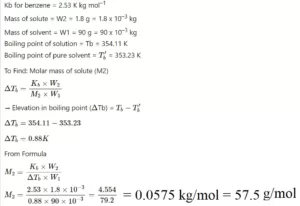Que 19. Write the hybridization and geometry of [Fe(CN)6]3- ion on the basis of Valence Bond Theory.
Ans 19.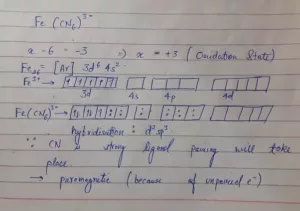OR

(i). Predict the number of unpaired electrons in the tetrahedral [MnBr4]2- ion and its magnetic moments.
(ii). [NiCl4]2- is paramagnetic while [Ni(CO)4] is diamagnetic though both are tetrahedral why?
Ans 19. (i)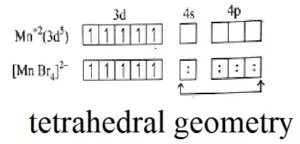Ans (ii). In [NiCl4]2- Ni is in +2 oxidation state with the configuration 3d8 4s0. Cl ion being weak ligand it cannot pair up the electrons in 3d orbitals. Hence it is paramagnetic. In [Ni (CO)4] Ni is in zero oxidation with the configuration 3d8 4s2. In the presence of CO ligand, the 4s electrons shift to 3d to pair up 3d electrons. Thus there is no unpaired electron present. Hence it is paramagnetic.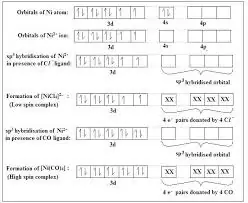Solved Question Paper Chemistry

Que 20. For a reaction, the rate law expression is represented as follows: Rate = k [A][B]1/2
(i). Interpret whether the reaction is elementary or complex. Give a reason to support your answer.
(ii). Write the units of the rate constant for this reaction if the concentration of A and B are expressed in moles/L.
Ans 20. (i) The reaction is a complex reaction. Order of reaction is 1.5.
Molecularity cannot be 1.5, it has no meaning for this reaction. The reaction occurs in steps, so it is a complex reaction.
(ii) Units of k are mol-1/2 L1/2 s-1

OR

In a reaction between A and B the initial rate of reaction (r0) was measured for different initial concentrations of A and B as given below

 A/mol/L 0.20 0.20 0.40 B/mol/ L 0.30 0.10 0.05 r0/molL‐ s‐ 5.07×10-5 5.07×10-5 1.43×10-4

What is the order of reaction with respect to A & B?
Ans 20. Let order of reaction wrt A is x & wrt B is y
R1=K [A]x.[B]y
R1= K(0.20)x .(0.03)y = 5.07×10‐5
R2 = K(0.20)x .(0.10)y = 5.07×10‐5
On Solving Eq. Y = 0
R1 = K(0.20)x .(0.03)y =5.07×10‐5
R3 = K(0.40)x.(0.05)y = 14.3×10‐5
On solving x = 1.5 So order of reaction w.r.t. A is 1.5 and w.r.t. B is 0.

Que 21. Write the mechanism of dehydration of Ethanol to yield Diethylether.
Ans 21.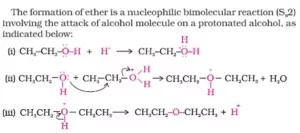OR

Explain how does the -OH group attach to a carbon of benzene ring activate it towards electrophilic substitution.
Ans 21. -OH is a group showing +R effect. It has two lone pairs. When it is attached to the carbon of benzene ring, its lone pair is donated to the ring (i.e, electrons are shifted towards the ring). These electrons now get engaged in Resonance, and hence there is a development of negative charge in Ortho and Para positions.
Electrophiles are positively charged groups, so they attack the positions containing the negative charge. And, since the ortho and para positions develop a negative charge, Electrophilic substitution is at ortho and para positions. So, we can conclude that –OH group is o-p directing.Solved Question Paper Chemistry

Que 22. For a first-order reaction show that time required for 99% completion is twice the time required for the completion of 90% of the reaction.
Ans 22. For a first-order reaction, the time required for 99% completion is
t= 2.303/k Log 100/100-99
= 2.303/k Log 100
= 2x 2.303/k
For a first order reaction, the time required for 90% completion is
t= 2.303/k Log 100/100-90
= 2.303/k Log 10
= 2.303/k
Therefore, t= 2t2
Hence, the time required for 99% completion of a first-order reaction is twice the time required for the completion of 90% of the reaction.

Que 23. The following haloalkanes are hydrolysed in presence of aq KOH.
(i) 2- Chlorobutane                  (ii) 2-chloro-2-methylpropane
Which of the above is most likely to give a racemic mixture? Justify your answer.
Ans 23. The racemic mixture will be given by 2-chlorobutane as it is an optically active compound.  When 2-chlorobutane undergoes SN1 reaction, both front and rear attack is possible, resulting in a racemic mixture.

Que 24. Draw the structures of the following: (i) SF4 (ii) XeF
Ans 24.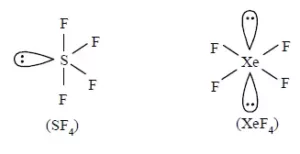Que 25. A compound is formed by two elements X and Y. The element Y forms ccp and atoms of X occupy octahedral voids. What is the formula of the compound?
Ans 25. Since atoms of Y forms CCP (cubic close packing) which is analogous to FCC (face centred cubic)
So, the effective number of atoms of Y in lattice =4
Number of octahedral voids = Number of atoms forming CCP =4
So, the effective number of atoms of X in the lattice =4
Now, X: Y ⇒ X: Y
4:4       1:1
So, the formula of the compound is XY

Solved Question Paper Chemistry

SECTION C

Q.No 26 -30 are Short Answer Type II carrying 3 marks each.

Que 26. (i) For the first row of transition metals, the E0 values are:-
E0 values   V            Cr             Mn             Fe               Co                 Ni            Cu
M2+/M     -1.18        -0.91          -1.18       -0.44          -0.28             -0.25        +0.34
(i) Explain the irregularity in the above values.
(ii) Why do transition elements show variable oxidation states?
Ans 26. (i) The E0 (M2+/M) values are not regular which can be explained by the irregular variation of ionization energy and sublimation energy of Mn due to half-filled orbitals.
(ii) Due to small energy difference between (n-1) d & ns orbital as a result both (n-1)d & ns electrons take part in bond formation.

OR

For M2+/M and M3+/M2+ systems, the E0 values for some metals are as follows:
Cr2+/Cr (-0.9V), Cr3+/Cr2+ (-0.4V), Mn2+/Mn (-1.2V), Mn3+/Mn2+ (+1.5V),
Fe2+/Fe (-0.4V), Fe3+/Fe2+ (+0.8V). Use this data to comment upon:
(i) The stability of Fe3+ in acid solution as compared to Cr3+ or Mn3+.
(ii) The ease with which iron can be oxidized as compared to a similar process for either chromium or manganese metal.
Ans 26. (i) E0 for Cr3+/Cr2+ is -0.4V  i.e. negative, this means Cr3+ ions in the solution cannot be reduced to Cr2+ easily i.e. Cr3+ is stable. As Mn3+/Mn2+ is +1.5V i.e positive means Mn3+ can easily reduced to Mn2+ ions in comparison to Fe3+ ions. Thus relatively stability of these ions is  Mn3+ < Fe3+ < Cr3+
(ii) The oxidation potentials for the given pairs will be +0.9V, +1.2V and 0.4V. Thus, the order of their getting oxidized will be in the order Mn > Cr > Fe.

Que 27. Account for the following:
(i) pKb of aniline is more than that of methylamine.
(ii) Ethylamine is soluble in water whereas aniline is not.
(iii) Aniline does not undergo Friedel-Crafts reaction.
Ans 27. (i)  Aniline undergoes resonance and as a result, the electrons on the N-atom are delocalized over the benzene ring. Therefore, the electrons on the N-atom are less available to donate. On the other hand, in the case of methylamine (due to the +I effect of methyl group), the electron density on the N-atom is increased. As a result, aniline is less basic than methylamine. Thus, the pKb of aniline is more than that of methylamine.
(ii) Ethylamine is soluble in water whereas aniline is not: Ethylamine when added to water forms intermolecular H−bonds with water. Hence, it is soluble in water. But aniline does not undergo H−bonding with water to a very large extent due to the presence of a large hydrophobic −C6Hgroup. Hence, aniline is insoluble in water.
(iii) Friedel-Crafts reaction is carried out in the presence of AlCl3. But AlCl3 is acidic in nature, while aniline is a strong base. Thus, aniline reacts with AlCl3 to form a salt (as shown in the following equation). Due to the positive charge on the N-atom, electrophilic substitution in the benzene ring is deactivated. Hence, aniline does not undergo the Friedel-Crafts reaction.

OR

(i) Write structures and IUPAC names of the amide which gives propanamine by Hoffmann bromamide reaction.
(ii) Write short notes on diazotization.
(iii) Give one chemical test to distinguish between Methylamine and dimethylamine.
Ans 27. (i) Propanamine contains three carbons. Hence, the amide molecule must contain four carbon atoms. Structure and IUPAC name of the starting amide with four carbon atoms is(ii) Aromatic primary amines react with nitrous acid (HNO2) at low temperatures (273-278 K) to form diazonium salts. This conversion of aromatic primary amines into diazonium salts is known as diazotization. For example, on treatment with  NaNO2 and HCl at 273−278 K, aniline produces benzenediazonium chloride, with NaCl and H2O as by-products.(iii) Methylamine and dimethylamine can be distinguished by the carbylamine test.
Carbylamine test: Aliphatic and aromatic primary amines on heating with chloroform and ethanolic potassium hydroxide form foul-smelling isocyanides or carbylamines. Methylamine (being an aliphatic primary amine) gives a positive carbylamine test, but dimethylamine does not.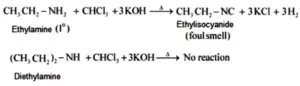Solved Question Paper Chemistry

Que 28. Differentiate primary, secondary and tertiary structure of the protein.
Ans 28. In Primary Structure, a specific sequence of an amino acid is present which is joined by covalent bonds.
Secondary Structure is responsible for the shape of a protein. α-helix and β-pleated in which polypeptide chains have peptide bonds.
Tertiary Structure represents the overall folding of the polypeptide chain and give rise to the fibrous or globular molecular shape.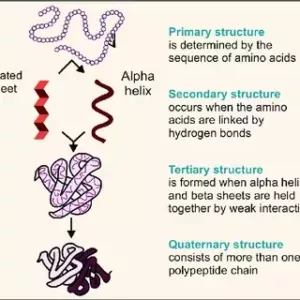Que 29. The density of chromium is 7.2gcm-3. If the unit cell is a cubic with a length of 289pm, determine the type of unit cell (Atomic mass of Cr=52 u and NA = 6.022×1023 atoms mol-1).
Ans 29.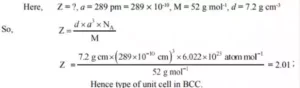Solved Question Paper Chemistry

SECTION – D

Que 30. Account for the following:-
(i) He is used in diving apparatus.
(ii) Noble gases have very low boiling points.
(iii) ICl is more reactive than I2.
Ans 30. (I) It is not soluble in the blood even under high pressure.
(ii) Being monoatomic they have weak dispersion forces.
(ii) I-Cl bond is weaker  than l-l bond

Que 31. (i) When Conc. H2SO4 was added to an unknown salt present in a test tube, a brown gas (A) was evolved. This gas intensified when copper turnings were added in to test tube. On cooling gas (A) changed into a colourless gas (B).
(a) Identify the gases ‘A’ and ‘B’
(b)Write the equations for the reactions involved
(ii) Arrange the following in the increasing order of the property mentioned.
(a) HOCl, HClO2, HClO3, HClO4 (Acidic strength)
(b) HF, HCl, HBr, HI (Acidic strength)
Ans 31.  (i) The brown gas (A) is nitrogen dioxide (NO2​). Salt reacts with conc. Sulphuric acid to form brown fumes of nitrogen dioxide.
MNO3​ + H2​SO4 ​→ MHSO4 ​+ HNO3
4HNO3​ → 4NO2​↑ + O2​ + 2H2​O
The fumes intensify when copper turnings are added.
3Cu + 8HNO3 ​→ 3Cu(NO3​)2 ​+ 2NO↑ + 4H2​O
2NO + O2​ → 2NO2​
At low temperature, odd electron, brown coloured NO2​ gas dimerises to colourless N2​O4​. NO2​ is paramagnetic and N2​O4​ is diamagnetic.
2NO2​ ⇌​ N2​O4
(ii) (a) Acidic strength: HOCl < HClO2 < HCIO3 < HCIO4
Reason: Oxygen is more electronegative than chlorine. With an increase in the number of O atoms attached to Cl, more electrons are pulled away from the O−H bond and becomes weaker. This increases the acid strength.
(b) Acidic strength: HF < HCl < HBr < HI
Reason: Down the group the electronegativity decreases and the size increases. Thus, it becomes easy to lose H+ ion. This causes an increase in acidic strength down the group.

OR

(i) Complete the following equations.
(a) XeF4 + H2O → 4 Xe + 2 XeO3+ 24 HF + 3O2
(b) AgCl(s) + NH3 (aq) → [Ag(NH3)2]Cl (aq)
(c) NaCl + MnO2 + 4H2SO4 → MnCl2 + 4 NaHSO4 + 2H2O + Cl2
(ii) (a) How is XeOF4 prepared? Draw its structure.
Ans (ii). Preparation of XeOF4
It can be prepared using XeF6​. The partial hydrolysis of XeF6​gives XeOF4​.
XeF6 ​+ H2​O ⟶ XeOF4 ​+ 2 HF
XeOF4​  has octahedral geometry due to sp3d2 hybridisation. One transposition is occupied by a lone pair giving pyramid shape to the molecule. Hence the molecular shape of XeOF4​ is square pyramidal.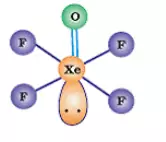Solved Question Paper Chemistry

Que 32. (i) An organic compound (A) (C8H16O2)was hydrolysed with dilute sulphuric acid to give a carboxylic acid (B)and alcohol (C). Oxidation of (C) with chromic acid produced (B). (C) on dehydration gives but-1-ene.Identity A,B,C.
Ans 32. (i) An organic compound A with molecular formula C8H16O2 gives a carboxylic acid (B) and alcohol (C) on hydrolysis with dilute sulphuric acid. Thus, compound A must be an ester. Further, alcohol C gives acid B on oxidation with chromic acid. Thus, B and C must contain an equal number of carbon atoms.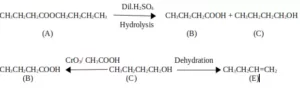(ii) Give reasons for the following:
(a) Cyclohexanone forms cyanohydrin in good yield but 2,2,6-trimethyl cyclo-hexanone does not.
Ans 32. (ii) (a)  In 2,2,6 trimethyl cyclohexanone there is the steric hindrance of 3 methyl groups, It does not form cyanohydrin in good yield.
(b) Carboxyl group in Benzoic acid is meta directing.
Ans. (b)  In benzoic acid, the Carboxyl group is meta directing because it is electron-withdrawing. There is a +ve charge on ortho and para positions. Electrophilic substitution takes place at meta-position.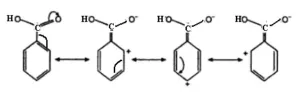OR

(i) An organic compound (A) with molecular formula C8H8O forms an orange-red precipitate with 2,4 DNP reagent and gives yellow precipitate on heating with iodine in the presence of sodium hydroxide. It neither reduces Tollen’s or Fehling’s reagent nor does it decolourise bromine water or Baeyer’s reagents. On drastic oxidation with chromic acid.it gives a carboxylic acid (B) having molecular formula C7H6O2. Identify the compounds (A) and (B).
Ans 32. (i)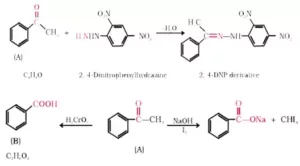(ii) Account for the following:-
(a) Aldehydes have a lower boiling point than corresponding alcohols.
(b) Methanal undergo Cannizzaro’s reaction.
Ans 32. (ii) a). The boiling point of aldehydes is lower than alcohols due to the absence of intermolecular hydrogen bonding. Alcohol contains an OH group allowing for hydrogen bonding which is stronger than other intermolecular forces.
(b). Because it does not possesses α-hydrogen atom

Que 33. (i) A voltaic cell is set up at 25°C with the following half-cells, Al3+ (0.0010 M) and Ni2+ (0.50 M). Write an equation for the reaction that occurs in the cell when the cell generates an electric current and determine the cell potential. (Given E °Ni 2+ /Ni = – 0.25V, E°Al3+/Al = -1.66V).
Ans 33. (i). At anode: Al(s) → Al3+ (aq) +3e] x 2
At cathode: Ni2+ (aq) + 2e →Ni (s)]x3
2Al (s) +3 Ni2+ (aq) → 2 Al3+ (aq) + Ni(s)
Here n = 6,
[Al3+] = 0.001M = 1× 10–3M, [Ni2+] = 0.5M
Correct formula
Correct answer and unit Ecell = 1.46 V
(ii) Electrolysis of KBr (aq) gives Br2 at anode but KF (aq) does not give F2. Give reason.
Ans 33. (ii). Oxidation takes place at the anode. Now higher the oxidation potential, easier to oxidize. The oxidation potential of Br-, H2O, F- are in the following order.

OR

(i) Calculate emf of the following cell at 298K
Zn/Zn2+ (104 M) || Cu2+ (10-2M)/Cu
Given E0 Zn2+/Zn = – 0.76V, E0Cu2+/Cu = +0.34V
Ans 33 (i). Cell reaction is as follows. Zn(s) + Cu2+→ Zn2+ + Cu(s)
n=2       T=298K
Ecell = (E0Cu2+/Cu – E0Zn2+/Zn)  – 0.0591/n log[zn+2]/Cu+2]
= 0.34V – (-0.76) – 0.02955 log 10-4/10-2
= 1.10 – 0.02955V log 10-2
= 1.10V+2 × 0.02955V
= 1.10V + 0.0591 V
= 1.1591V
(ii) Name the factor on which emf of a cell depends.
Ans 33 (ii). emf of a cell depends on the following factor:
a). Nature of electrodes
b). Temperature
c). The concentration of half cells.

Solved Question Paper Chemistry